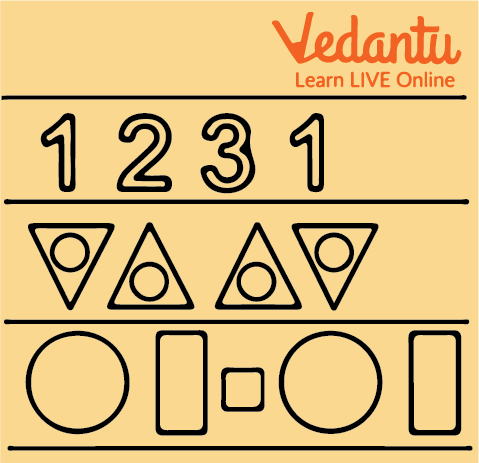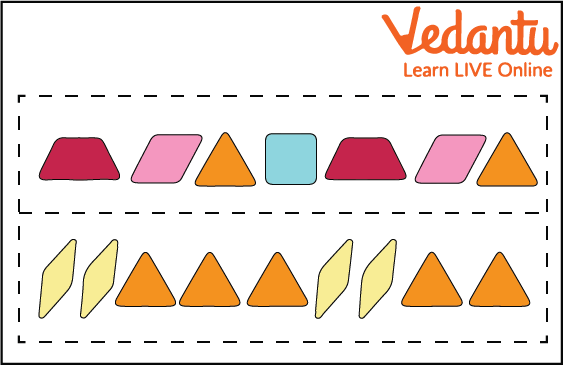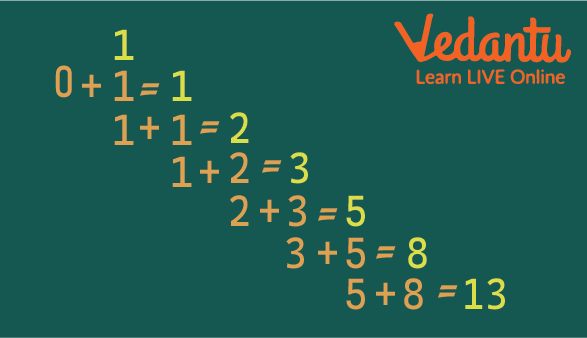Courses
Courses for Kids
Free study material
Free LIVE classes
More

# Patterns for KidsLIVE
Join Vedantu’s FREE Mastercalss

## What are Patterns?

A pattern is a series or chain that usually repeats itself. Colour, activity, form, number and other patterns that we see in our daily lives are examples of patterns. They might be finite or infinite and can be tied to any event or object. In Mathematics, a pattern is a sequence of integers that are connected to each other according to a specified rule. These rules specify how to compute or solve issues. In a sequence of 3, 6, 9, 12, _, each number increases by 3, therefore, the number after 12 is 15.

The pattern is a sequence that follows a specific order and a common relationship between each and every member. In Maths, there are several forms of patterns, such as numbers and symbols, picture patterns, logic patterns, word patterns and so on. The number pattern is the most widely employed since children are familiar with even numbers, odd numbers, skip counting and other concepts that aid in comprehending these patterns.Example of Different Types of Patterns

## Finite and Infinite Patterns

• The pattern in which the first and last members of the pattern are known, the pattern is said to be a finite pattern.

Example of Patterns: In pattern 3, 6, 9, 12, and 15, the first term is 3 and the last term is 15.

• An infinite pattern is a sequence in which the first member is known but the last member is unknown.

Example: In pattern 3, 6, 9, 12, 15, 18…, the first term is 3, but where the pattern will stop is not known.

## Rules of Pattern

To create a specific pattern, you need to consider a specific set of rules. To apply these rules, you must first understand the nature of the sequence and the difference between two consecutive numbers given in the pattern. The following rule, which takes a little guesswork, works for the whole pattern.

Patterns can be formed using three categories.

• Repeating Pattern - A type of pattern which follows the same rule in the whole pattern is known as a repeating pattern. Usually, these are letters and shapes.

• Growing Pattern - When numbers are arranged in ascending order in a growth pattern, or when each number is greater than the previous number, such a pattern is called a growing pattern. Example: 2, 6, 10….

• Shrinking Pattern - In this pattern, the numbers are sorted in descending order, or each number is less than the previous one. Example: 9, 8, 7, 6….Repeating Pattern in Shapes

## Types of Patterns in Maths

• The Arithmetic Pattern - Usually referred to as the algebraic pattern is a number series formed by addition or subtraction to construct a number sequence connected to each other. If we are given two or more integers from the series, we may use addition or subtraction to identify the mathematical pattern and to find the missing number in a given sequence.

• A Geometric Pattern - is a numerical sequence focused on multiplication and division. If we have two or more numbers in the sequence, we can easily discover the unknown numbers in the patterns by applying multiplication and division.

• The Fibonacci Pattern - is a number sequence in which each number is produced by combining the two preceding numbers together. The number sequence begins with 0 and 1.Fibonacci Pattern Example

## Solved Examples

1. Find the missing term in the series 2, 8, 14, 20, __?

a. 24

b. 26

c. 30

d. 32

Ans: 26

Explanation: There is an addition of 6 in each number. By adding 6 to 20 you can find the missing term. Thus, the missing term will be 26.

2. 1, 2, 3, 4, 5………. this series is

a. Finite

b. Infinite

c. None of the above

Ans: Infinite

Explanation: Since there is only the first term known but the last term is unknown, so the series is an infinite series.

3. What is the next term of series 22, 20, 18, 16, _?

a. 14

b. 12

c. 15

d. 18

Ans: 14

Explanation: Since the series follows a decreasing order. Every term decreases by 2. So, the next term after 16 will be 14.

## Conclusion

There are several forms of Maths patterns. They might be ascending or descending, multiples of a certain number, or a series of even or odd numbers. Seeing a pattern prompts us to think about and determine the rule that will allow the pattern to continue. Patterns can be made up of forms, objects and colours in addition to numbers.

Last updated date: 23rd Sep 2023
Total views: 92.1k
Views today: 2.92k

## FAQs on Patterns for Kids

1. What exactly is a Number Pattern?

A number pattern depicts the link between two or more numbers. It is described as a collection of integers that follow a particular pattern or sequence. For example, in the above sequence: 5, 10, 15, 20, 25, 30,..., each term in the pattern is created by adding 5 to the previous term.

2. What are the different types of patterns?

There are three different types of patterns namely shape pattern, letter pattern and number pattern. The pattern displayed by the different shapes is the shape pattern. If letters of the alphabet show a pattern, then it is a letter pattern and when numbers are present in different patterns, then it is a number pattern.

3. What does a growing pattern mean?

When numbers are arranged in ascending order in a growth pattern, or when each number is greater than the previous number, such a pattern is called a growing pattern. Example: 2, 6, 10….

4. Give an example of patterns in nature for kids.

Some of the patterns that can be seen in nature are waves formed by the sea or ocean, sand dunes, spots and stripes present on animals, and branching patterns of trees.

5. Why is the infinite series called infinite?

In infinite series, you don't know how long this series continues and the last term of the series is not known. That is why such series are called infinite series.